## COVERING GROUND [DAY 2]

### AREA OF A TRIANGLE & PARALLELOGRAM

Investigate the area of a triangle and area of a parallelogram to generalize a formula for each.

## Intentionality & Unit Overview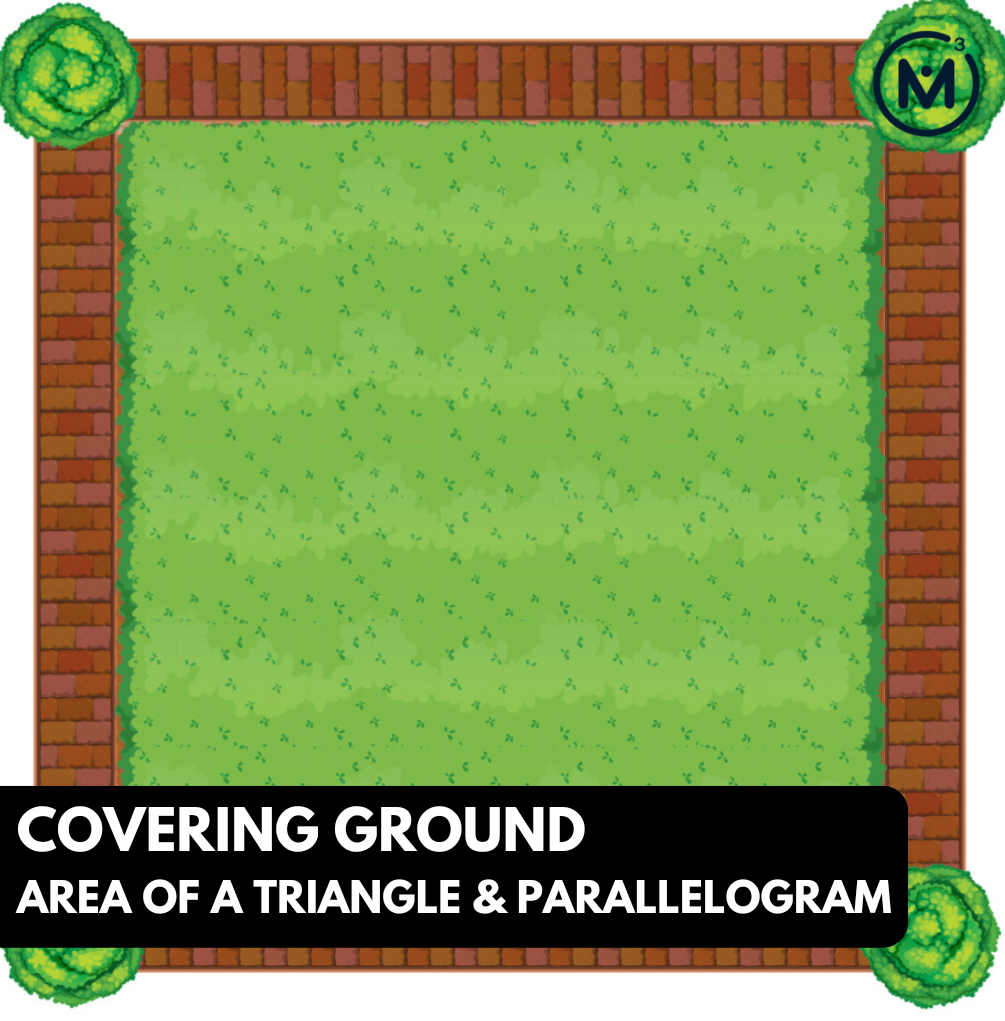### Access each lesson from this unit using the navigation links below

Students will continue exploring the relationship between rectangles and triangles to figure out how to calculate the area of a triangle.

## Intentionality…

The purpose of the Day 2 activities is to reinforce key concepts from Day 1. Students will engage in a math talk and will have an opportunity to complete independent purposeful practice. The math talk and purposeful practice serve to develop a deeper understanding of the following big ideas.

• The area of a rectangle can be determined by multiplying the length of its base by the length of its height;
• The area of a rectangle is used to determine the area formulas for other polygons;
• Using “base” and “height” rather than “length” and “width” can support student understanding when determining the area formulas for triangles and all quadrilaterals;
• A parallelogram is any quadrilateral with two sets of parallel sides;
• A rectangle is a parallelogram;
• The relationships between rectangles, parallelograms, and triangles can be used to determine area formulas;
• Any triangle can be doubled to create a parallelogram;
• Congruent shapes have the same size and shape, but not necessarily the same orientation.

## Math Talk

Present the following triangles. Students are asked to calculate the area of each triangle in square units. Encourage students to share their thinking. Represent the student thinking symbolically.

For example, if a student said they found the area of the rectangle and then divided the area by 2, this could be represented symbolically as:

area =  $$\frac{(base\hspace{5px}x\hspace{5px}height)}{2}$$

area =  $$\frac{10 units\hspace{5px}x\hspace{5px}8 units}{2}$$

area = 40 square units

Alternatively, if a student says that they multiplied the base by half the height, this could be represented symbolically as:

area = base x ($$\frac{1}{2}$$ height)

area = 10 units x 4 units
area = 40 square units

Familiarise students with the use of variables when working with formulas.

## Visual Math Talk Prompt #1

Show students the following visual math talk prompt and be prepared to pause the video where indicated:

Calculate the area of each triangle in square metres.
Craft a convincing argument without the use of a calculator.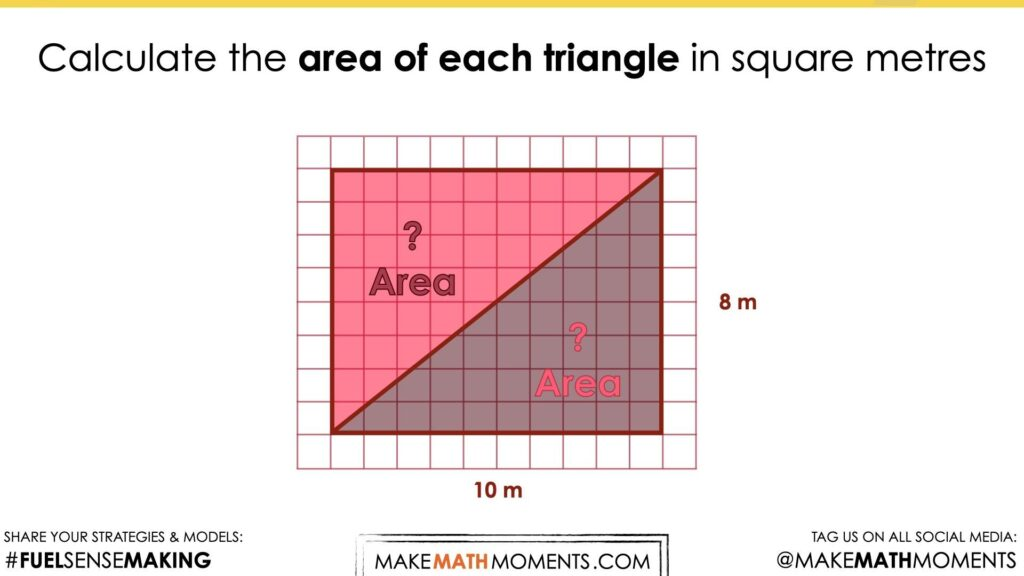As you may immediately notice, we have given students two congruent triangles and have conveniently arranged them such that together, they create a rectangle. This is intentional to ensure that all students explicitly see that every rectangle can be partitioned diagonally into two congruent triangles. From this visual math talk prompt, we hope to reiterate that partitioning any rectangle diagonally into two congruent triangles will result in each triangle having half of the area of the rectangle.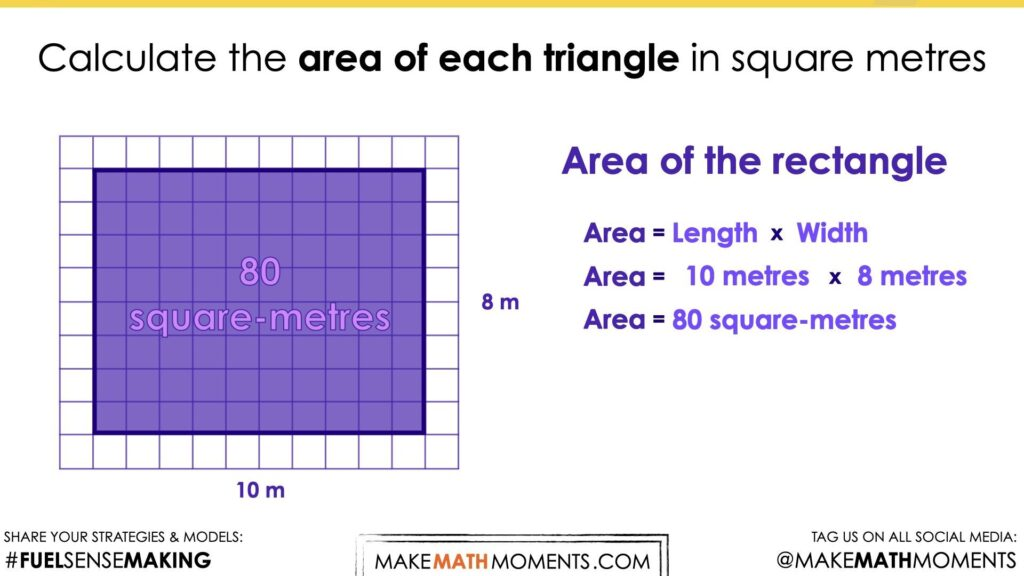Therefore, it may feel intuitive to some students to first find the area of the rectangle and simply half that quantity to determine the area of each of the resulting triangles.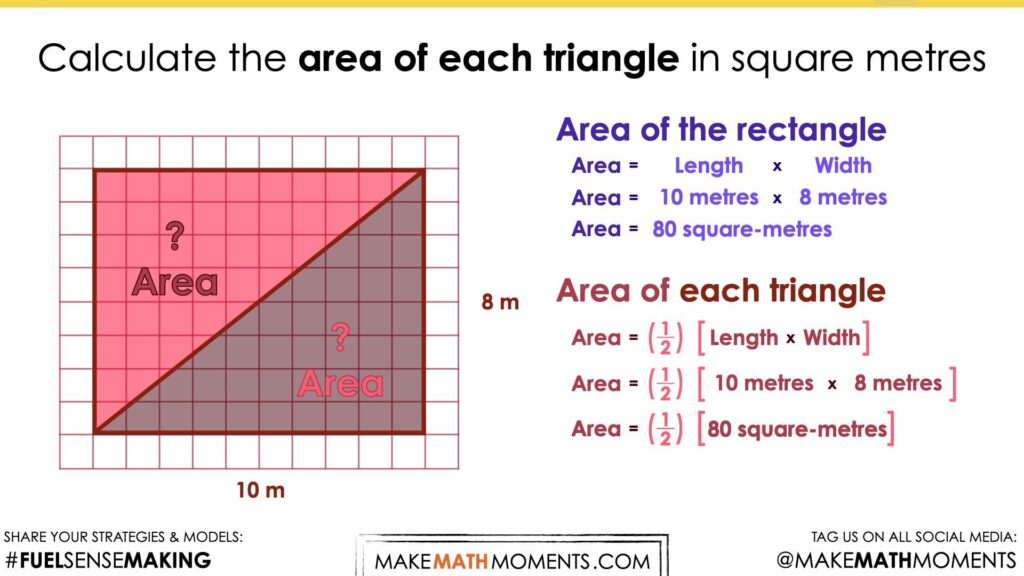While some students may perform the operation of taking half of the area of the rectangle (or dividing the area by 2), it is likely that many will not naturally write their thinking down symbolically as you see on the right of the screen. The goal here is not necessarily to ensure that all students “copy down” the formula, but rather so they can make a connection between the intuitive actions they had completed to find the area of the triangles to the symbolic representation of that same thinking.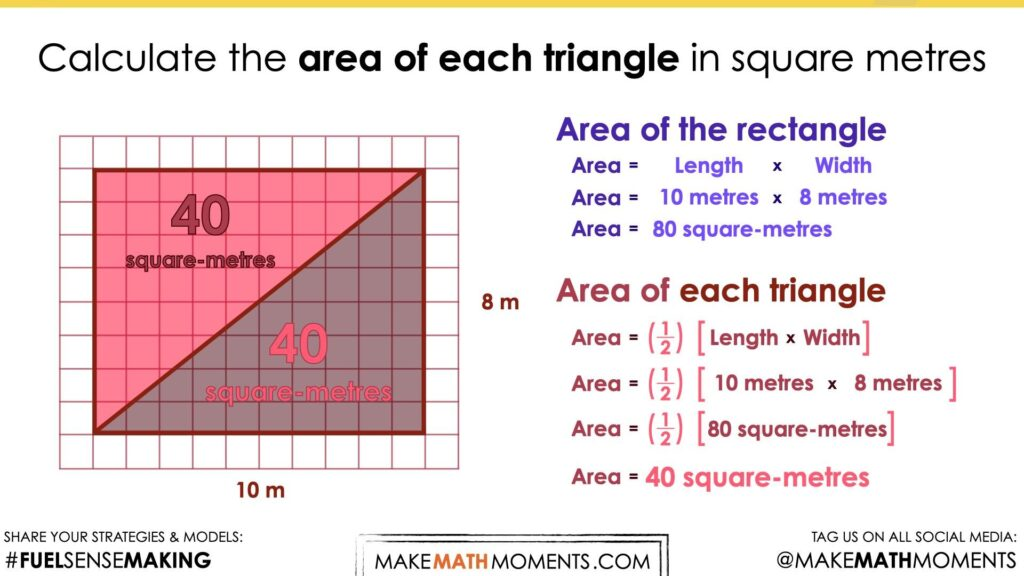It is important to explicitly highlight that the two triangles that were created by partitioning the rectangle diagonally result in congruent triangles as this may not be obvious to all students immediately.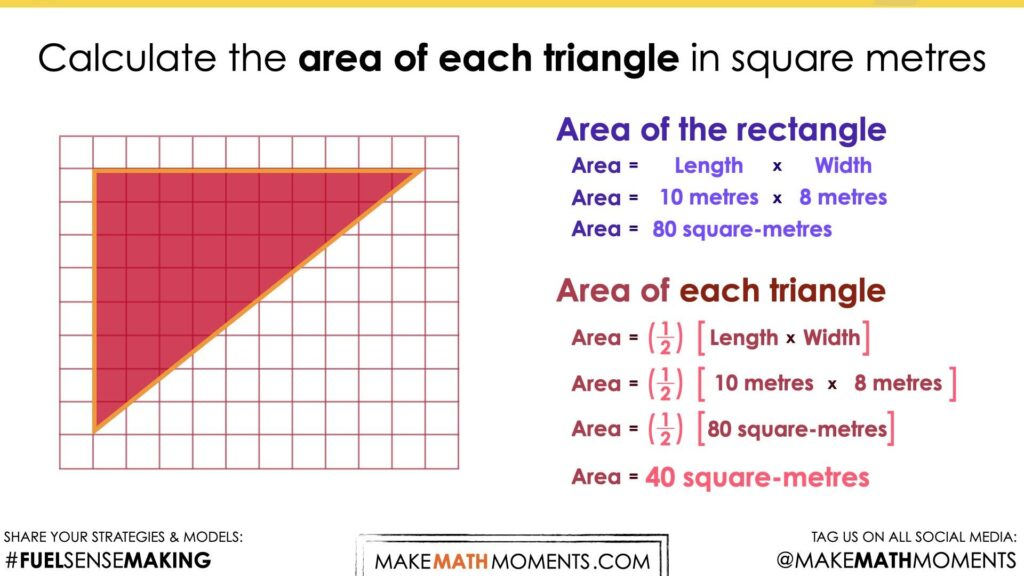It could also be helpful to show students that despite taking half of the area of the rectangle (or dividing the area by 2) revealing the area of each congruent triangle, the same operation can be done to determine the area of other congruent shapes that result when partitioning the original rectangle in half. For example, horizontally partitioning the rectangle into halves allows us to complete the same mathematical operation to reveal the same area.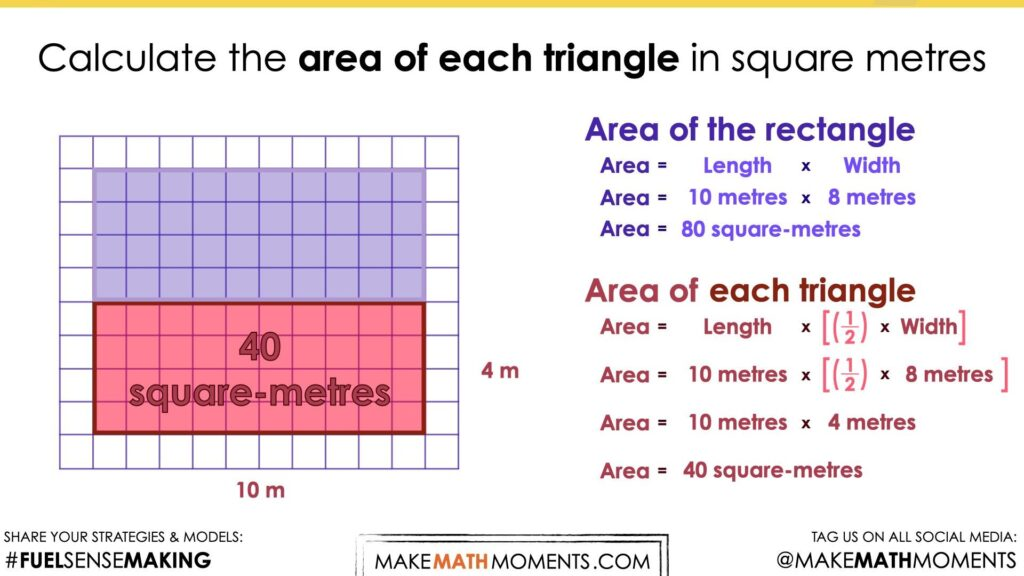This idea helps to highlight the fact that representing mathematical thinking of area symbolically can strip away information about how the units of area are arranged (i.e.: as a rectangle, a triangle, or some other shape).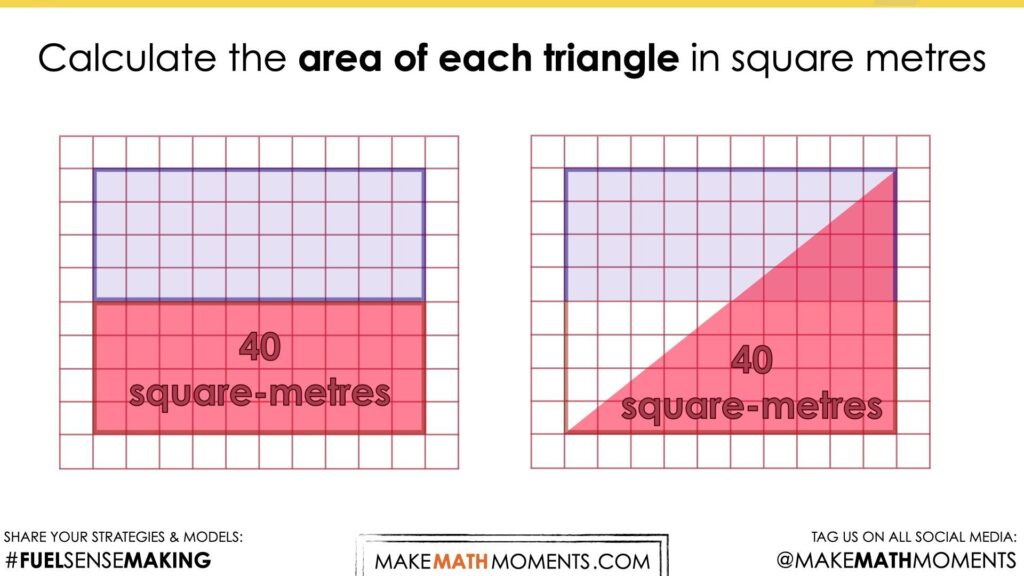## Visual Math Talk Prompt #2

Show students the following visual math talk prompt and be prepared to pause the video where indicated:

Calculate the area of each triangle in square metres.
Craft a convincing argument without the use of a calculator.Here we give students another opportunity to apply their understanding of how the area of a triangle relates to the area of a rectangle in order to continue working towards the emergence of a generalization and a formula.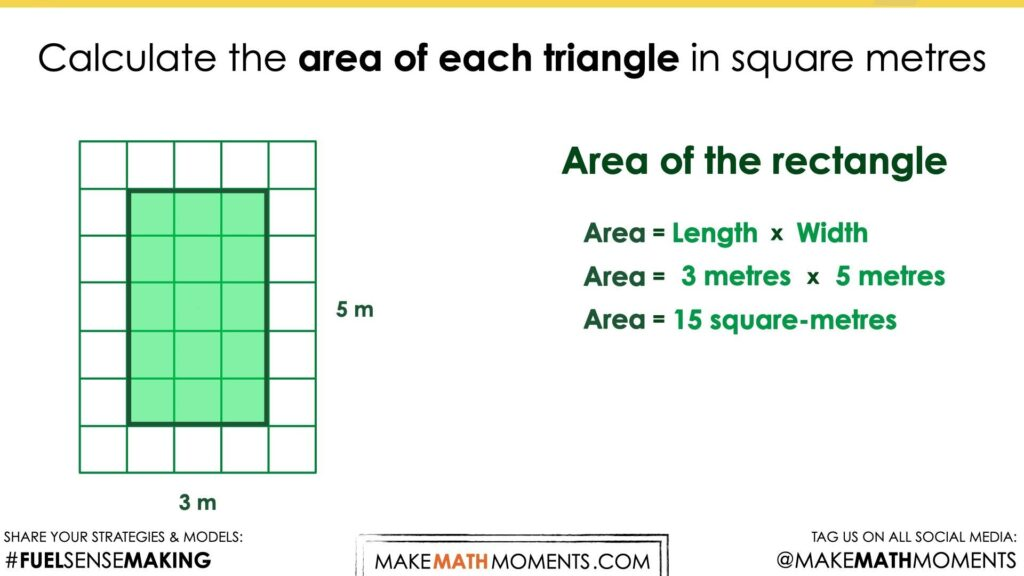In this situation, all students can access determining the area of the rectangle by skip counting or leveraging a known multiplication fact (i.e.: 3 x 5).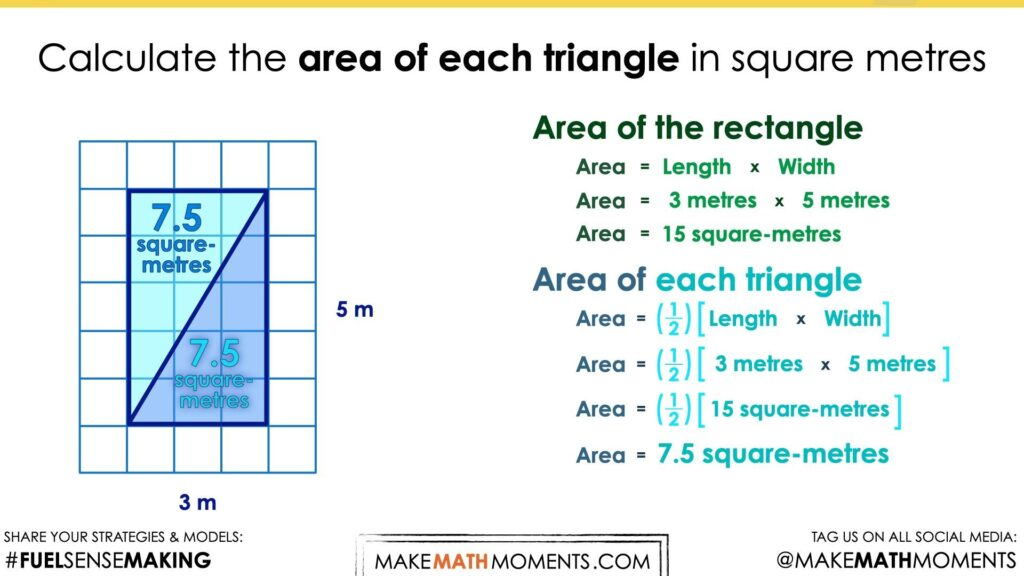Applying the same logic as number talk prompt #1, students can then half (or divide the area by 2) in order to reveal the area of each triangle.

Again, it can be helpful to show that dividing the area of a rectangle by 2 (or halving the area) reveals half the quantity of area units, but does not reveal information about how the area is organized (i.e.: what shape(s) are formed).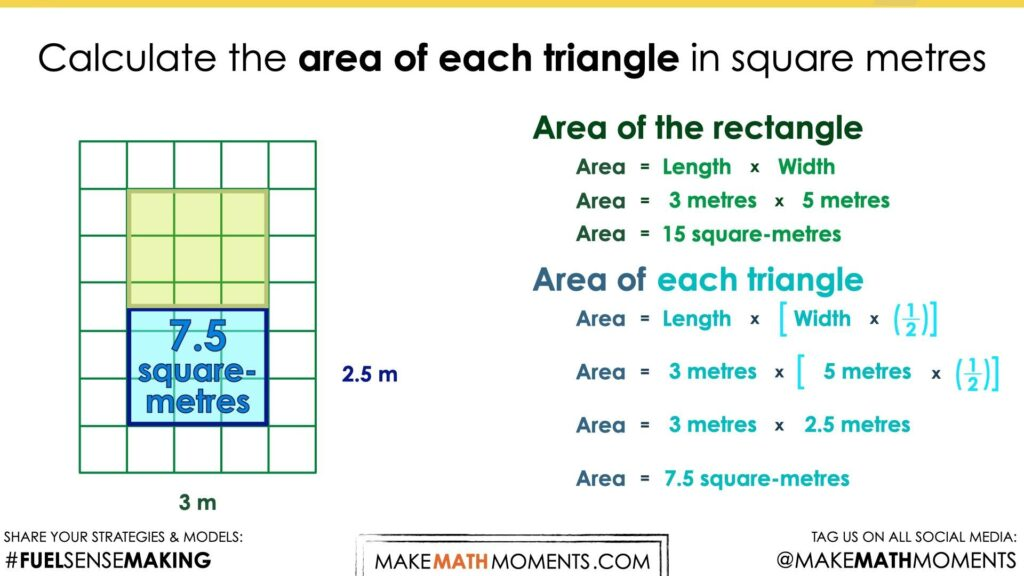## Visual Math Talk Prompt #3

Login/Join to access the entire Teacher Guide, downloadable slide decks and printable handouts for this lesson and all problem based units.

## Purposeful Practice

Login/Join to access the entire Teacher Guide, downloadable slide decks and printable handouts for this lesson and all problem based units.

## Questions: Area of a Triangle

Login/Join to access one (1) additional Math Talk Prompt as well as the Teacher Guide, downloadable slide decks and printable handouts for this lesson and all problem-based units.

Login/Join to access the entire Teacher Guide, downloadable slide decks and printable handouts for this lesson and all problem based units.

## Educator Discussion Area

Login/Join to access the entire Teacher Guide, downloadable slide decks and printable handouts for this lesson and all problem based units.

## Explore Our 60+ Problem Based Units

This Make Math Moments Lesson was designed to spark curiosity for a multi-day unit of study with built in purposeful practice, number talks and extensions to elicit and emerge strategies and mathematical models.

Dig into our other units of study and view by concept continuum, grade or topic!